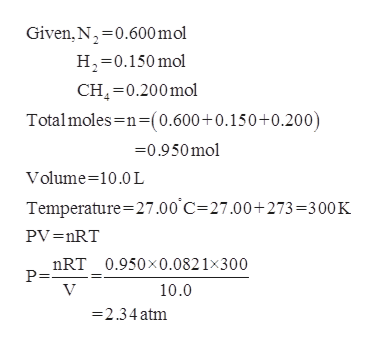# A gas mixture contains 0.600  mol of N2, 0.150  mol of H2, and 0.200  mol of CH4. Calculate the pressure of the gas mixture and the partial pressure of each constituent gas if the mixture is in a 10.0  L vessel at 27.00°C.

Question

A gas mixture contains 0.600  mol of N2, 0.150  mol of H2, and 0.200  mol of CH4. Calculate the pressure of the gas mixture and the partial pressure of each constituent gas if the mixture is in a 10.0  L vessel at 27.00°C.

check_circle

Step 1

The pressure of the gas mixtur...help_outlineImage TranscriptioncloseGiven, N 0.600 mol H 0.150mol CH 0.200mol Totalmoles n-(0.600+0.150+0.200) 0.950mol Volume 10.0 L Temperature 27.00 C 27.00+ 273 =300 K PV =nRT nRT_0.950x0.0821x300 P= V 10.0 =2.34atm fullscreen

### Want to see the full answer?

See Solution

#### Want to see this answer and more?

Solutions are written by subject experts who are available 24/7. Questions are typically answered within 1 hour.*

See Solution
*Response times may vary by subject and question.
Tagged in

### Physical Chemistry